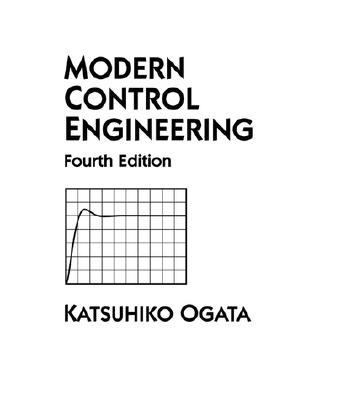191757

9780130609076

# Modern Control Engineering\$40.93
\$3.95 Shipping
List Price
\$150.00
Discount
72% Off
You Save
\$109.07

• Condition: Good
• Provider: Ergodebooks Contact
• Provider Rating:
81%
• Ships From: Multiple Locations
• Shipping: Standard
• Comments: Buy with confidence. Excellent Customer Service & Return policy. Ships Fast. 24*7 Customer Service.30-day money back guarantee

Most renters respond to questions in 48 hours or less.
The response will be emailed to you.
• ISBN-13: 9780130609076
• ISBN: 0130609072
• Edition: 4
• Publication Date: 2001
• Publisher: Prentice Hall

Ogata, Katsuhiko

### SUMMARY

This book presents a comprehensive treatment of the analysis and design of control systems. It is written at the level of the senior engineering (mechanical, electrical, aerospace, and chemical) student and is intended to be used as a text for the first course in control systems. The prerequisite on the part of the reader is that he or she has had introductory courses on differential equations, vector-matrix analysis, circuit analysis, and mechanics. The main revision made in the fourth edition of the text is to present two-degrees-of-freedom control systems to design high performance control systems such that steady-state errors in following step, ramp, and acceleration inputs become zero. Also, newly presented is the computational (MATLAB) approach to determine the pole-zero locations of the controller to obtain the desired transient response characteristics such that the maximum overshoot and settling time in the step response be within the specified values. These subjects are discussed in Chapter 10. Also, Chapter 5 (primarily transient response analysis) and Chapter 12 (primarily pole placement and observer design) are expanded using MATLAB. Many new solved problems are added to these chapters so that the reader will have a good understanding of the MATLAB approach to the analysis and design of control systems. Throughout the book computational problems are solved with MATLAB. This text is organized into 12 chapters. The outline of the book is as follows. Chapter 1 presents an introduction to control systems. Chapter 2 deals with Laplace transforms of commonly encountered time functions and some of the useful theorems on Laplace transforms. (If the students have an adequate background on Laplace transforms, this chapter may be skipped.) Chapter 3 treats mathematical modeling of dynamic systems (mostly mechanical, electrical, and electronic systems) and develops transfer function models and state-space models. This chapter also introduces signal flow graphs. Discussions of a linearization technique for nonlinear mathematical models are included in this chapter. Chapter 4 presents mathematical modeling of fluid systems (such as liquid-level systems, pneumatic systems, and hydraulic systems) and thermal systems. Chapter 5 treats transient response analyses of dynamic systems to step, ramp, and impulse inputs. MATLAB is extensively used for transient response analysis. Routh's stability criterion is presented in this chapter for the stability analysis of higher order systems. Steady-state error analysis of unity-feedback control systems is also presented in this chapter. Chapter 6 treats the root-locus analysis of control systems. Plotting root loci with MATLAB is discussed in detail. In this chapter root-locus analyses of positive-feedback systems, conditionally stable systems, and systems with transport lag are included. Chapter 7 presents the design of lead, lag, and lag-lead compensators with the root-locus method. Both series and parallel compensation techniques are discussed. Chapter 8 presents basic materials on frequency-response analysis. Bode diagrams, polar plots, the Nyquist stability criterion, and closed-loop frequency response are discussed including the MATLAB approach to obtain frequency response plots. Chapter 9 treats the design and compensation techniques using frequency-response methods. Specifically, the Bode diagram approach to the design of lead, lag, and lag-lead compensators is discussed in detail. Chapter 10 first deals with the basic and modified PID controls and then presents computational (MATLAB) approach to obtain optimal choices of parameter values of controllers to satisfy requirements on step response characteristics. Next, it presents two-degrees-of-freedom control systems. The chapter concludes with the design of high performance control systems that will follow a step, ramp, or acceleration input without steady-state error. The zero-placement methodOgata, Katsuhiko is the author of 'Modern Control Engineering', published 2001 under ISBN 9780130609076 and ISBN 0130609072.

You can find lots of answers to common customer questions in our FAQs

View a detailed breakdown of our shipping prices